# Maximum diversification portfolio

Spreading out investments to reduce risk is one of the most important considerations when constructing and investment portfolio. One approach is maximum diversification. It is a portfolio approach that tries create a portfolio that is as diversified as possible. In particular, the strategy tries to maximize the diversification ratio. As such, the maximum diversification approach is a risk-based allocation approach that does not take into account expected returns. The approach is aimed at obtaining a well-diversified portfolio. On this page, we discuss how a maximum diversification portfolio approach should be implemented.

## Maximum diversification definition

To explain maximum diversification, we will need some mathematics. First, we discuss how we will measure the level of diversification in a portfolio. Let X1,, Xn be a set of securities. Let V be the covariance matrix of these assets, and let C be the corresponding correlation matrix.

Next, let’s define a vector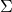that contains the asset volatilities.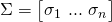Finally, the portfolio weights are P = [w1, …, wn], with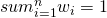. In that case, the diversification ratio of the portfolio P can be defined as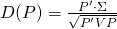In other words, the diversification ratio is defined as the ratio of the weighted average of volatilities divided by the portfolio volatility. In practice, a manager will impose a set of linear constraints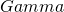to the weights of the portfolio. One example of a set of constraints is a long-only constraint. This means that all the weights should be positive.

The portfolio, under the set of constraints that the manager chooses, that maximizes the value D(P) is the most diversified portfolio.

## maximum diversification portfolio optimization

Unlike the minimum variance portfolio approach, the maximum diversification approach does not have a closed form solution. To perform the maximum diversification portfolio optimization, we require software that can maximize (in practice minimize) the objective function. In this case the objective function is the diversification ratio of the portfolio. In particular, we try to determine the set of weights w that minimizes the diversification ratio.

## Summary

We discussed the maximum diversification portfolio approach. The approach determines the weights, subject to linear constraints, that maximizes the diversification ratio.